#### You may also like### I'm Eight

Find a great variety of ways of asking questions which make 8.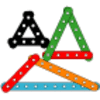### Let's Investigate Triangles

Vincent and Tara are making triangles with the class construction set. They have a pile of strips of different lengths. How many different triangles can they make?### Noah

Noah saw 12 legs walk by into the Ark. How many creatures did he see?

# Getting the Balance

##### Age 5 to 7 Challenge Level:

Here we have a balance for you to work on:

Full Screen Version

It is a number balance, sometimes it's called a "Balance Bar" and sometimes an "Equalizer".
It has weights like these;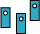These weights are hung below the numerals. It balances equal amounts, for example, with $10$ on one side and $2$ and $8$ on the other we have;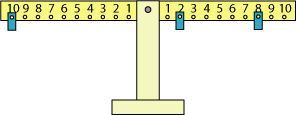If you like this idea try "Number Balance ", then return here.
Now this challenge is about getting the balance.

Rule : All the while you can only have one weight at each numeral on the balance.

Let's start by saying that on one side of the balance, place two weights and keep them there. Make it balance by placing $3$ weights on the other side (remember only one at each numeral!).

So you might start with an $8$ and a $3$ on one side, and find you have to have something like these for it to balance: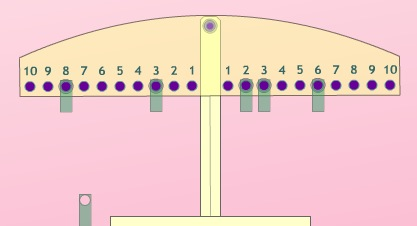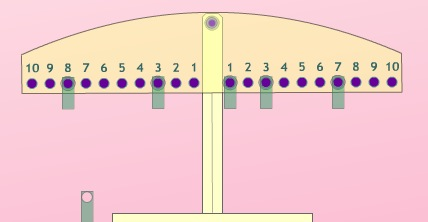So choose your two places on one side and find many different balance places on the other side.

When you've done all you can, it might be an idea to choose another (maybe higher) pair of numbers for one side and find all the ways of placing $3$ weights on the other side.

Are you recording your results? If so, how?